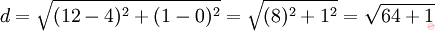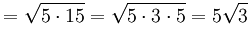# Given (12, 1) and (4, 0), find the midpoint, distance, slope, and equation of the line.

(Last Updated On: December 13, 2017)

### Problem: Given these pairs of points, (12, 1) and (4, 0), find the midpoint, distance, slope, and equation of the line.### Solutions:

• To find the midpoint, average the x coordinates and y coordinates. The midpoint is• To find the (always zero or positive) distance, use the formula• To find the slope, use the formula• The equations of the line are

Method 1:Plug in one known point (say, (4, 0) ) and the calculated slope.Now plug b and m into the line equation:

•Method 2:Plug in one known point (say, (12, 1) ) and the calculated slope.•### NOTE:

For any Questions, Clarifications and any violent Reactions use the comment section below. I’ll be much willing to assist you.

credit: Todd
Rate this: